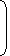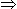# Tricks to solve Speed Time and Distance Problems for Competitive Exams#### TIME,DISTANCE AND SPEED

Distance covered per unit time is called SPEED

i,e., speed=distance/time

the above equation can be rearranged as

time=distance/speed

distance=speed*time

#### 1.km/hr to m/sec conversion

 zkm/hr =z x 5/18m/sec

#### 2.m/sec to km/hr conversion

 z m/sec =z x 18/5km/hr

#### WORKSAMPLES:

1. A person crosses a 600 m long street in 5 minutes. What is his speed in km per hour?
 A. 3.6 B. 7.2 C. 8.4 D. 10

SOLUTION WITH EXPLANATION:

 Speed =600m/sec. 5 x 60

= 2 m/sec.

Converting m/sec to km/hr (see important formulas section)

 =2 x 18km/hr 5

= 7.2 km/hr

2.If a person walks at 14 km/hr instead of 10 km/hr, he would have walked 20 km more. The actual distance travelled by him is:
 A. 50 km B. 56 km C. 70 km D. 80 km

SOLUTION WITH EXPLANATION:

Let the actual distance travelled be x km.

 Then, x = x + 20 10 1414x = 10x + 2004x = 200x = 50 km.

Correct! Wrong!

Correct! Wrong!

Correct! Wrong!

Correct! Wrong!

#### 5.A man on tour travels first 160 km at 64 km/hr and the next 160 km at 80 km/hr. The average speed for the first 320 km of the tour is?

Correct! Wrong!

TIME MANAGEMENT IS LIFE MANAGEMENT
yeahhhh!!! you have done a great work